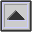TRAFFIC NOISE INDEX (TNI)

A method devised in the U.K. to measure annoyance responses to motor vehicle noise. It takes into account the traffic flow and therefore functions similarly to the NOISE AND NUMBER INDEX used to evaluate aircraft noisiness.

Traffic levels are monitored over a 24-hour period and TNI is derived by combining the NOISE LEVELs exceeded in dBA 10 per cent and 90 per cent of the time. This takes into account the very noisy vehicles weighted against the general traffic noise.

The TNI is computed from the formula:

TNI = 4 . (L10 - L90) + (L90 - 30) (dB)

where L10 and L90 are the A-weighted DECIBEL levels exceeded 10% and 90% of the time respectively (i.e. the peak and ambient levels respectively).

For example, if L10 is 59 dBA and L90 is 44 dBA, the TNI would be 74 dB, a level found in social surveys to produce less than 3% dissatisfaction, and therefore a level suggested for planning purposes with regard to determining an optimum distance for dwellings from roadways.

Ref.: F.J. Langdon and W.E. Scholes, The Traffic Noise Index: A Method of Controlling Noise Nuisance, Building Research Station Current Papers 38168, April 1968, pp. 2-3.

home